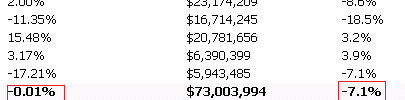# New to QlikView

Discussion board where members can get started with QlikView.

Announcements
Leverage your QlikView investment to modernize BI – see how! Join Group
cancel
Showing results for
Did you mean:
HighlightedCreator III

## Wrong % total in pivot table

Hi,

Am getting wrong grand total in pivot table.Previously it is good .Thanks..

Tags (1)
1 Solution

Accepted Solutions
HighlightedMVP

## Re: Wrong % total in pivot table

Could you explain how you got to -7.91% ? Or why do you call -0.012% wrong and -7.91% correct?

It seems to me that you are calculating something like a growth rate per dimension value.

IMHO, I would expect to see in the total line the growth rate calculated from the total aggregates, and not summing up or averaging the growth rate of the dimension lines.

Hence, -0.012% might be correct, at least I don't see an issue with using the expression total here instead of an aggregation-of-rows.

Regards,

Stefan

4 Replies
HighlightedMVP

## Re: Wrong % total in pivot table

Can you provide more details? What is the expression you are using? What changed that the totals changed from what was expected? What is the expected output?

HighlightedCreator III

## Re: Wrong % total in pivot table

Hi,

Dimension : =if(KEY3='Appliances' or KEY3='Electronics' or KEY3='Furniture' or  KEY3='Mattress' or                  KEY3='Office',KEY3)

Expression:1

(Sum({<DATE={'\$(Vyesterday)'},Indicator={1}>}Dollars)

-

Sum ({<DATE={'\$(vDayLY)'},Indicator={1}>} Dollars))

/

Sum ({<DATE={'\$(vDayLY)'},Indicator={1}>} Dollars)

Expression:2

(Sum ({<DATE= {">=\$(vMonthStart)<=\$(Vyesterday)"},Indicator={1}>} Dollars)-Sum ({<DATE= {">=\$(vMonthStartLY)<=\$(vPrevYesterday)"},Indicator={1}>} Dollars))

/

Sum ({<DATE= {">=\$(vMonthStartLY)<=\$(vPrevYesterday)"},Indicator={1}>} Dollars)

Desired O/P:

 2.00% -11.35% 15.48% 3.17% -17.21%

Total =-7.91%

But it gives -0.012%.

Note: Didn't change any chart properties it working good since log time.

Thanks..

HighlightedMVP

## Re: Wrong % total in pivot table

Can you try this:

Sum(Aggr((Sum ({<DATE= {">=\$(vMonthStart)<=\$(Vyesterday)"},Indicator={1}>} Dollars)-Sum ({<DATE= {">=\$(vMonthStartLY)<=\$(vPrevYesterday)"},Indicator={1}>} Dollars))

/

Sum ({<DATE= {">=\$(vMonthStartLY)<=\$(vPrevYesterday)"},Indicator={1}>} Dollars), KEY3))

HighlightedMVP

## Re: Wrong % total in pivot table

Could you explain how you got to -7.91% ? Or why do you call -0.012% wrong and -7.91% correct?

It seems to me that you are calculating something like a growth rate per dimension value.

IMHO, I would expect to see in the total line the growth rate calculated from the total aggregates, and not summing up or averaging the growth rate of the dimension lines.

Hence, -0.012% might be correct, at least I don't see an issue with using the expression total here instead of an aggregation-of-rows.

Regards,

Stefan